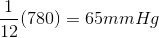# AP Chemistry : Partial Pressure

## Example Questions

### Example Question #1 : Partial Pressure

Each of the following compounds are contained in a closed container with a volume of 100mL. Which of the following will exert the most pressure?

60g CO2

54g F2

50g O2

46g N2

40g H2

40g H2

Explanation:

The pressure exerted is dependent on molar concentration only (not mass). As the containers are all the same volume, the container with the most moles will exert the most pressure. 60g of 44g/mole CO2 gives 1.4mol, 50g of O2 gives 1.4mol, 46g N2 gives 1.6mol, and 54g F2 gives 1.4mol, 40g H2 gives 40mol.

### Example Question #1 : Partial Pressure

A mixture of three gases in a balloon is able to generate 2 atm of pressure.  The identies and amounts of each gas are as follows.  2 moles of O2, 3 moles of N2, 5 moles of H2.

What is the partial pressure of Oxygen gas?

2 atm

1.6 atm

0.4 atm

0.5 atm

4 atm

0.4 atm

Explanation:

Partial pressure is the amount of pressure that is the result of one gas. This is calculated by mutiplying the mole fraction of the gas by the overall pressure.  The mole fraction is calculated as the moles of the compound of interest divided by the total amount of moles present.  2 moles O2/ 2 moles O2 + 3 moles N2 + 5 moles H2 = 2/10 = mole fraction of O2.  2/10 multiplied by the total pressure gives us the answer as 0.4 atm.

### Example Question #37 : Gases

A mixture of gases is present in a container. The total pressure on the container is 12atm. Six moles of one of the gases accounts for nine atmospheres of pressure. What is the molar fraction of the remaining gases in the container?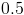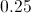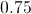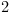Explanation:

There are a couple of things to consider in this problem. First, the problem asks for the molar fraction of the remaining gases in the mixture. As a result, we do not need to know how many different gases are in the mixture, since they all have the same effect on the pressure in the container. Second, the number of moles that one gas accounts for is not relevant since the question is asking to find the molar fraction for the remaining gases. Knowing this, we can find the molar fraction for the described gas, and then subtract it from the total in order to find the remaining molar fraction. The equation for partial pressure is written as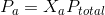.

Since we know how much pressure one gas accounts for, we can solve for the molar fraction of that particular gas.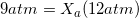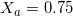In other words, the known gas accounts for 75% of the gas molecules in the container. This means that the remaining gases must account for 25% of the gas molecules in the container.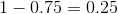So, the molar fraction for the remaining gases is 0.25.

### Example Question #1 : Partial Pressure

If a container holds 1mol of hydrogen, 2.5mol of helium, and 2mol of oxygen at a total pressure of 4atm, what is the partial pressure of the oxygen gas?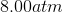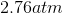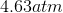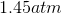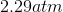Explanation:

According to Dalton's law of partial pressures, when two or more gases are in one container without chemical interaction, each behaves independently of the others. The partial pressure of oxygen can, therefore, be found by multiplying the molar fraction of oxygen in the container by the total pressure of the three gases.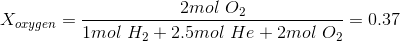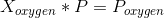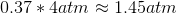### Example Question #39 : Gases

A glass container holds a mixture of two gases. Gas A exerts a pressure of 5atm on the container.

If there are twice as many moles of gas A as there are moles of gas B in the container, what is the total pressure in the container?

The pressure is unaffected by the gas of lower concentration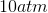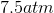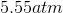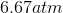Explanation:

This is an equation which requires us to find the partial pressures of each gas in the container. The equation for partial pressure iswhereis the molar fraction of one of the gases. Since there are twice as many moles of gas A as there are gas B, we know that gas A accounts for 2/3 of the moles in the container.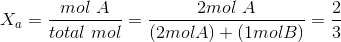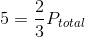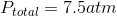### Example Question #40 : Gases

A gas mixture at equilibrium held over water at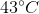contains 2.0 moles of oxygen, 9.0 moles of nitrogen, and 1.0 moles of water vapor. What is the vapor pressure of water at, if the total pressure exerted by the gases is 780mmHg?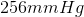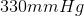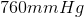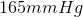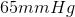The molar quantity of a gas is directly proportional to its partial pressure, so if we have a total of 12 moles of gas, and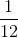of the gas sample is water vapor, then water vapor makes upof the total pressure. Thus to get the partial vapor pressure of any of the substances, we multiply the total vapor pressure by the proportion of that gas.